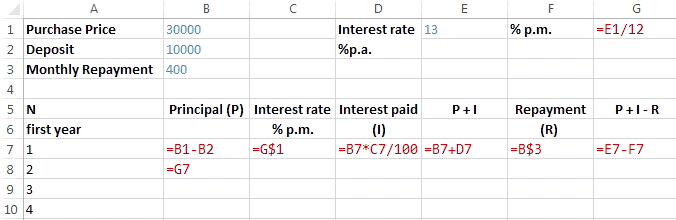Excel formula

Reducible Interest | # gomaths.net/4264         User submitted, thanks to Amanda M

Calculate the reducible interest on a car loan.

B7: `=B1-B2`, C7: `=G\$1`, D7: `=B7*C7/100`, E7: `=B7+D7`
F7: `=B\$3`, G7: `=E7-F7`, B8: `=G7`